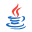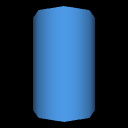## X3D Example Archives: VRML 2 Sourcebook, Chapter 19 Normals Shading

 Normal (perpendicular) vectors defined for individual points or polygons can change the shading of geometry. When shaded, the faces on a shape are obvious. Creating a smooth shape may require a large number of small faces. An alternative is to instead use smooth shading to create the illusion of a smooth shape, but with a smaller number of faces. The Normal node lists normal vectors to use for parts of a shape which can change shading. These are used as the value of the normal field, and normal index values select which normals to use (similar to color index values). Ordinarily the creaseAngle field controls faceted or smooth shading. Normals override the normal effect of the creaseAngle value. The normalPerVertex field selects normal per face/grid square or normal per coordinate. The NormalInterpolator node converts time values to normal values for animation effects. These X3D scenes are adapted directly from the original VRML 2.0 Sourcebook chapter examples. Also available: Introduction to VRML97 SIGGRAPH98 course notes.
9 X3D Models       X3D Model DescriptionsFigure 19 21 Normal Square A square face and four normals. Also see Figures 19.21 and 19.22 for comparison.Figure 19 22 Normal Square Shaded A square face with two normals pointing along the positive Z axis and two normals pointing along the positive X axis. Also see Figures 19.21 and 19.22 for comparison.Figure 19 23 Half Column A smooth-shaded half column using a crease angle. Compare Figures 19.23, 19.24a, 19.24b, 19.25a and 19.25b.Figure 19 24a Half Column Crease Angle A smooth-shaded half column using a crease angle. Compare Figures 19.23, 19.24a, 19.24b, 19.25a and 19.25b.Figure 19 24b Two Half Columns Crease Angle Seam A smooth-shaded half column using a crease angle - note seams where each smoothly shaded half-column meets. Compare Figures 19.23, 19.24a, 19.24b, 19.25a and 19.25b.Figure 19 25a Half Column Normals A smooth-shaded half column using a crease angle. Compare Figures 19.23, 19.24a, 19.24b, 19.25a and 19.25b.Figure 19 25b Two Half Columns Normals Seamless A smooth-shaded half column using a crease angle. Compare Figures 19.23, 19.24a, 19.24b, 19.25a and 19.25b.Figure 19 26 Normal Shaded Elevation Grid An elevation grid using a special shading effect.Figure 19 27 Square Face Animating Normals An elevation grid using a special shading effect.